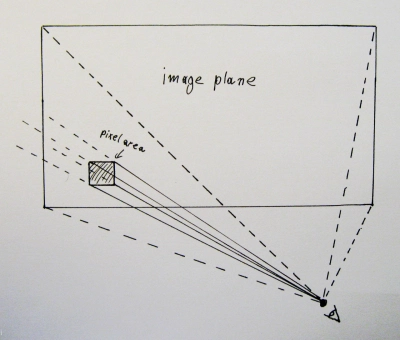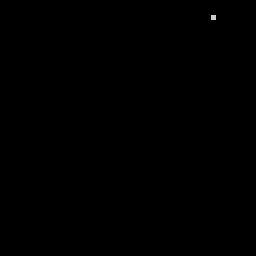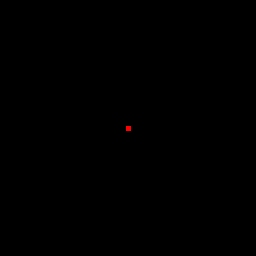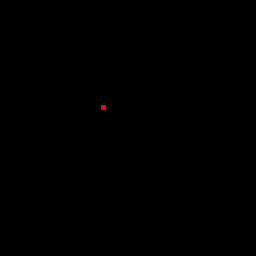Generating multiple sample positions per pixel

John Tsiombikas nuclear@member.fsf.org

3 November 2012

Anti-aliasing in ray tracing, requires casting multiple rays per pixel, to sample the whole solid angle subtended by the imaginary surface of each pixel, if we consider it to be a small rectangular part of the view plane (see diagram).It's obvious that to be able to generate multiple primary rays for each pixel, we need to have an algorithm that given the sample number, calculates a sample position within the area of the a pixel. Since it's trivial to map points in the unit square, onto the actual area of an arbitrary pixel, it makes sense to write this sample position generation function, so that it calculates points in the unit square.

The easiest way to write such a function would be to generate random points in the unit square, like this:

void get_sample_pos(float *pos)
{
pos = (float)rand() / (float)RAND_MAX - 0.5;
pos = (float)rand() / (float)RAND_MAX - 0.5;
}

The problem with this approach is that, even if our random number generator really has a perfectly uniform distribution, any finite number of sample positions generated, especially if that number is in the low tens, will probably not cover the area of the pixel in anything resembling a uniform sampling. Clusters are very likely to occur, leaving large areas of the pixel space unexplored by our rays. As the number of samples gets larger and larger, this problem is somewhat mitigated, but especially if we're not writting a path tracer, we’re usually dealing with anything between 4 to 20 rays per pixel, no more.

The following animation shows random sample positions generated by the code above. Even at about 40 samples, the left part of the pixel is inadequately sampled.Another approach is to avoid randomness. The following function gets the sample number as input, and calculates its position by recursively subdividing the pixel area, taking care to spread the samples of each recursion level around as much as possible instead of focusing on one quadrant at a time.

void get_sample_pos(int sidx, float *pos)
{
pos = pos = 0.0f;
get_sample_pos_rec(sidx, 1.0f, 1.0f, pos);
}

static void get_sample_pos_rec(int sidx, float xsz, float ysz, float *pos)
{
static const float subpt = {
{-0.25, -0.25}, {0.25, -0.25}, {-0.25, 0.25}, {0.25, 0.25}
};

/* base case: sample 0 is always in the middle, do nothing */
if(!sidx) return;

/* determine which quadrant to recurse into */
quadrant = ((sidx - 1) % 4);

get_sample_pos_rec((sidx - 1) / 4, xsz / 2, ysz / 2, pos);
}

And here's the animation showing that code in action (colors denote the recursion depth):This sampling is perfectly uniform, but it's still not ideal. The problem is that whenever we're sampling in a regular grid, no matter how fine that grid is, we will introduce aliasing. By breaking up each pixel into multiple subpixels like this we effectively increase the cutoff frequency after which aliasing occurs, but we do not eliminate it.

The best solution is to combine both techniques. We need randomness to convert aliasing into noise, which is much less perceptible by human brains trained by evolution to recognize patterns. But we also need uniform sampling to properly explore the whole area of each pixel.

So, we'll employ a technique known as jittering: first we uniformly subdivide the pixel into subpixels, and then we randomly perturb the sample position of each subpixel inside the area of that subpixel. The following code implements this algorithm:

void get_sample_pos(int sidx, float *pos)
{
pos = pos = 0.0f;
get_sample_pos_rec(sidx, 1.0f, 1.0f, pos);
}

static void get_sample_pos_rec(int sidx, float xsz, float ysz, float *pos)
{
static const float subpt = {
{-0.25, -0.25}, {0.25, -0.25}, {-0.25, 0.25}, {0.25, 0.25}
};

if(!sidx) {
/* we're done, just add appropriate jitter */
pos += (float)rand() / (float)RAND_MAX * xsz - xsz / 2.0;
pos += (float)rand() / (float)RAND_MAX * ysz - ysz / 2.0;
return;
}

/* determine which quadrant to recurse into */
quadrant = ((sidx - 1) % 4);

get_sample_pos_rec((sidx - 1) / 4, xsz / 2, ysz / 2, pos);
}

And here's the animation showing the jittered sample position generator in action:This was initially posted in my old wordpress blog. Visit the original version to see any comments.

Discuss this post

Back to my blog